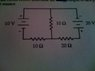# I'm having trouble with this circuit problem - Power

JMoore0150
The homework problem is attached as a .jpg file and it states:
"For this circuit, determine the Power Output of each Power Supply & the Power Conserved by Each Resistor. The multiple lines kind of threw me for a loop for the 10v & 20V. Thanks and any help is much appreciated.
Best,
Jimbo Moore

#### Attachments

•Circuit Problem C.jpg
22.1 KB · Views: 362

Queue
The homework problem is attached as a .jpg file and it states:
"For this circuit, determine the Power Output of each Power Supply & the Power Conserved by Each Resistor. The multiple lines kind of threw me for a loop for the 10v & 20V. Thanks and any help is much appreciated.
Best,
Jimbo Moore

Your attachment is awaiting approval. Perhaps you can host it somewhere else (such as photobucket) and we can help you a bit quicker.

Homework Helper
You need to write 2 loop equations for the inner loops, remembering to take the current through the middle resistor as the sum of the 2 currents.

Pick a direction for the current in each loop and then add voltages around the loop. They should add to 0 over any closed loop.

You will have 2 equations. And you will have 2 unknowns I1 and I2. Just solve.

JMoore0150
So where does the power output of the "power supply" and the power "Conserved" come into play with the equation? Why is there 2 ends to each voltage? That's throwing me off and so is trying to understand what exactly it is asking for. What directions do these 2 voltages cause throughout the circuit? Thanks!

JMoore0150
Also, is there any examples on here or elsewhere that anyone knows of that have vast similarities to this equation? Or if I am to search for it, what should my keywords be? Thanks again!

Homework Helper
As a first step don't you think you need to determine the current in the loops, so you can address the V*I of the voltage sources and the resistors?